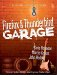Understanding Bayesian Filtering

 < Day Day Up >

 Shortly after his death in 1761, Reverend Thomas Bayes's (1702 1761) work in statistical distributions was published by his friend Richard Price. This work is now known as the Bayes Theorem. Almost 240 years later, Paul Graham published his paper "A Plan for Spam," which proposed using Bayes Theorem (in a slightly modified form, perhaps) to detect spam. To use Bayesian filtering, you must have a collection of spam emails (several thousand would be best) and a collection of emails that are not spam (again, a few thousand). These messages are then fed to the filter software, which creates lists of words in each category, spam and nonspam. Each word includes a count of how often it occurs (so a word such as Viagra might have a relatively high count in the spam messages but not be found in the nonspam messages). After these counts are created, they are plugged into an algorithm to compute the probability that they are an indicator of spam. Here's how it works: Compute the approximate percentage of spam emails. We'll call this percentage Pe.Take the 15 most interesting words. Graham defines interesting words as those with the score furthest from average.Compute the combined probability that these words are spam. Each probability is based on the values found in the cache of words and is based on how often these words are found in spam and how often they're found in nonspam.Compute the combined probably that these words are in a spam email. Graham provides the following formula for three words:Ps = xyz ÷ (x + y + z) + (1 x)(1 y)(1 z)This formula simply expands based on the number of words.Compute the combined probability that the 15 most interesting words might be found in any email (both spam and nonspam). We can call this probability Pa.Finally, the probability that a message is spam is computed using Ps, like so:Probability that an email is spam = Ps x Pe ÷ Pa Finally, we take this resulting probability and make a decision. Most systems flag an email as spam when the probability is 90% or greater. Empirical testing shows that few emails fall within the middle probabilities; most fall either at the higher end (and are spam) or at the lower end (and are not spam).
 < Day Day Up >Firefox and Thunderbird Garage
ISBN: 0131870041
EAN: 2147483647
Year: 2003
Pages: 245

Similar book on Amazon